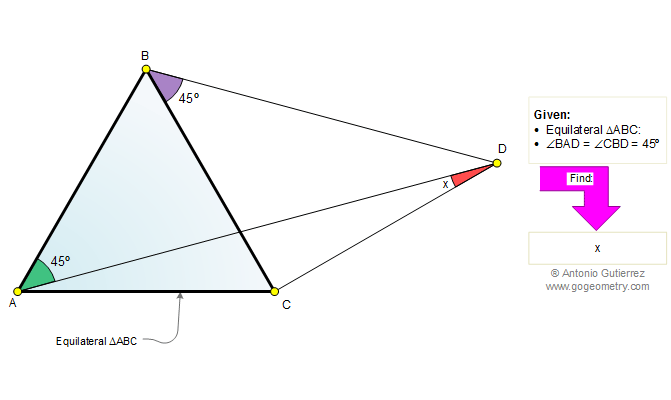Problem 504. Equilateral Triangle, Angles, 45, 60 Degrees. Level: High School, College, SAT Prep. The drawing shows a quadrilateral ABDC with an equilateral  triangle ABC. If the measure of angle BAD is 45 degrees and angle CBD is 45 degrees, find the measure of the angle ADC.Recent Additions